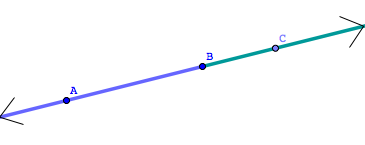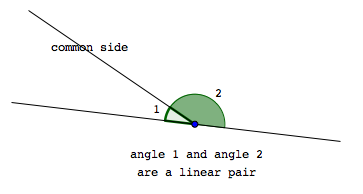﻿ Angles: Complementary, Supplementary, Vertical, and Linear Pairs
ANGLES: COMPLEMENTARY, SUPPLEMENTARY,
VERTICAL, and LINEAR PAIRS

For this web exercise, assume all angles are measured in degrees.

DEFINITIONS complementary angles, supplementary angles
Two angles are complementary   iff   the sum of their measures is $\,90^{\circ}\,$.

Two angles are supplementary   iff   the sum of their measures is $\,180^{\circ}\,$.

In particular:

• if $\,\angle 1\,$ and $\,\angle 2\,$ are complementary, then $\,m\angle 1+ m\angle 2 = 90^{\circ}\,$
• if $\,\angle 1\,$ and $\,\angle 2\,$ are supplementary, then $\,m\angle 1+ m\angle 2 = 180^{\circ}\,$

DEFINITION opposite rays
 Rays that: share a common endpoint, and point in opposite directions are called opposite rays.Note:   If three points are on a line with $\,B\,$ between $\,A\,$ and $\,C\,$, then $\,\overrightarrow{BA}\,$ and $\,\overrightarrow{BC}\,$ are opposite rays.

Recall that both $\,A{-}B{-}C\,$ and $\,C{-}B{-}A\,$ are notation for ‘$\,B\,$ is between $\,A\,$ and $\,C\,$’.

DEFINITION linear pair
 Two angles are a linear pair   iff they have a common side, and their other sides are opposite rays.Note:   If $\,\angle 1\,$ and $\,\angle 2\,$ are a linear pair, then $\,m\angle 1+ m\angle 2 = 180^{\circ}\,$.

DEFINITION vertical angles
 Two angles are vertical angles   iff   the sides of one angle are opposite rays to the sides of the other.Note:   Vertical angles are the ‘opposite angles’ that are formed by two intersecting lines.

Note:   If $\,\angle 1\,$ and $\,\angle 2\,$ are vertical angles, then $\,m\angle 1 = m\angle 2\$.

DEFINITION parallel lines
Two lines are parallel if and only if they lie in the same plane and do not intersect.

Parallel lines are studied in more detail in a future section, Parallel Lines.

The symbol ‘$\,\parallel\,$’ is used to denote parallel lines.

The sentence ‘$\,\ell\parallel m\,$’ is read as ‘$\,\ell \,$ is parallel to $\,m\,$’,
and is true precisely when line $\,\ell\,$ is parallel to line $\,m\,$.

DEFINITION perpendicular lines
Two lines are perpendicular if and only if they form a right angle.

The symbol ‘$\,\perp\,$’ is used to denote perpendicular lines.

The sentence ‘$\,\ell\perp m\,$’ is read as ‘$\,\ell \,$ is perpendicular to $\,m\,$’,
and is true precisely when line $\,\ell\,$ is perpendicular to line $\,m\,$.

EXAMPLE:
Question: Suppose that $\,\angle 1\,$ and $\,\angle 2\,$ are vertical angles.
Suppose that   $\,m\angle 1 = 7x-15\,$   and   $\,m\angle 2 = 2x+55\,$.
Find $\,x\,$.
Solution:
 $7x-15= 2x+55$ (vertical angles have equal measures) $5x-15=55$ (subtract $\,2x\,$ from both sides) $5x=70$ (add $\,15\,$ to both sides) $x=14$ (divide both sides by $\,5\,$)
Master the ideas from this section